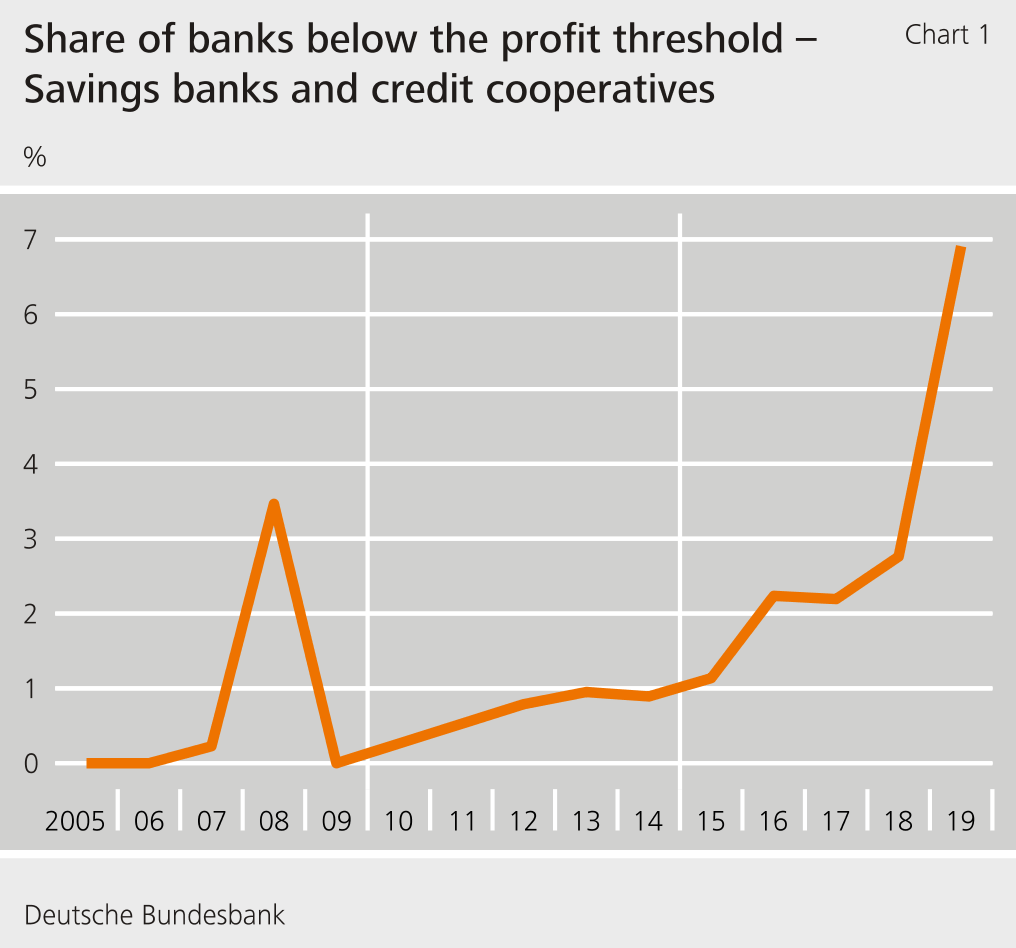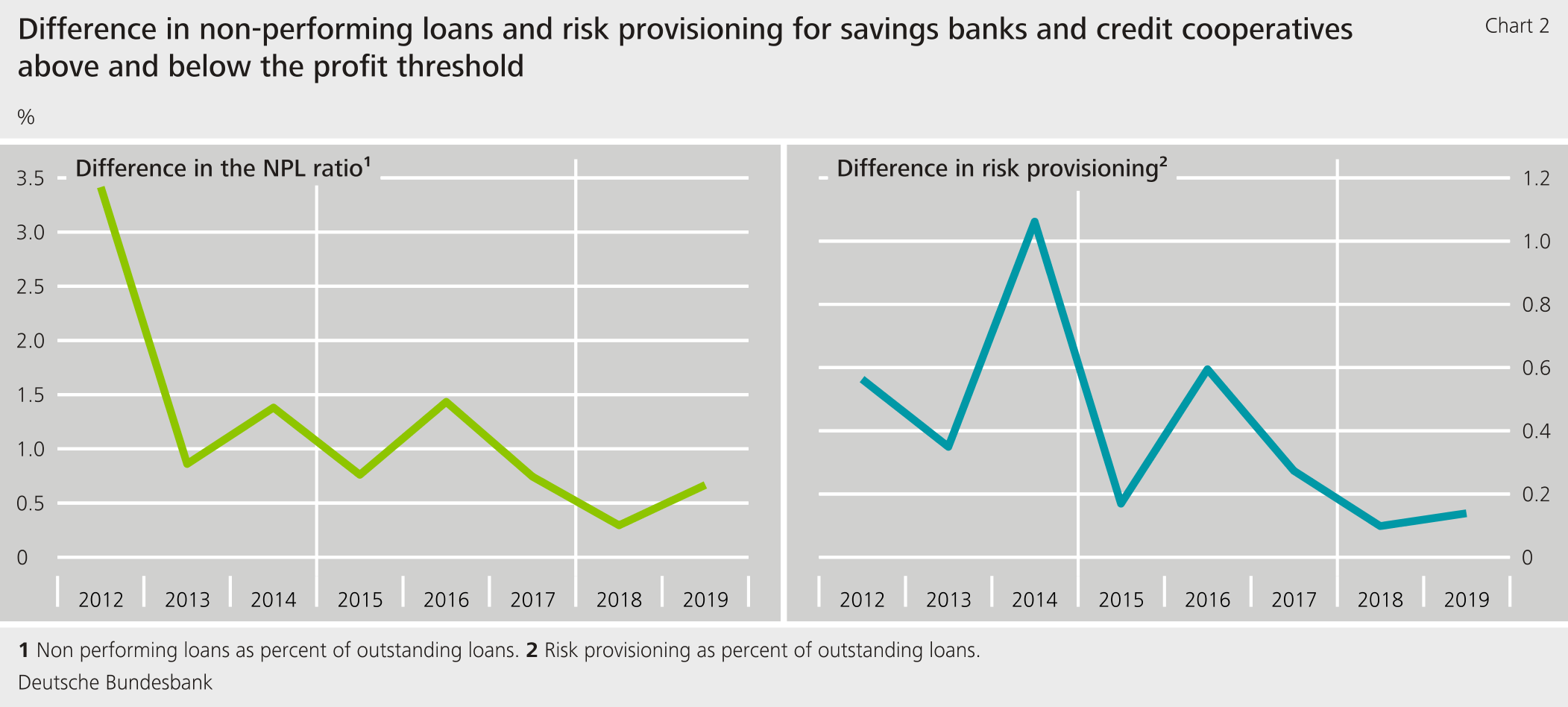# Banks with low profitability increasingly taking risks in the low interest rate environment Research Brief | 42nd edition – October 2021

Banks grant long-term loans funded by short-term customer deposits. This maturity transformation earns banks money because long-term interest rates are generally higher than short-term interest rates. At the same time, this exposes banks to the risk that interest rates will rise, forcing them to pay more for deposits in the short term already, whereas they only receive the higher interest on new loans. In the low interest rate environment which has prevailed over the past few years, the premium on assuming interest rate risk has trended downward. At the same time, banks with poor profitability have stepped up their maturity transformation and thus also this risk. This is potentially a sign of a search for yield.

Demand for an asset generally rises if the investor expects a higher return given a constant risk, i.e. if the risk premium rises. This is precisely the result produced, under certain conditions, by the theoretical model in our study, co-written with Max Teichert (Memmel, Seymen and Teichert, 2018). However, in contrast to conventional models in the literature, it also incorporates the opposite case, in which demand for the asset rises even though the expected return falls. We apply the theoretical model to banks’ decision to take increased interest rate risk by looking at banks as investors and interest rate risk as the asset. In the theoretical model, a bank with high gross profits is risk averse, i.e. it only takes higher interest rate risks if this is associated with a sufficient risk premium. As gross profits decline, the bank becomes increasingly risk-seeking, i.e. the relationship between the elevated interest rate risk and the premium on this risk becomes weaker and, if the gross profits are sufficiently low, the relationship even becomes negative: banks whose gross profits drop below a critical level take greater interest rate risk even though the risk premium falls.

Such behaviour is potentially a sign of a search for yield because, for instance, the remuneration of a given bank’s management is performance-related and so management therefore participates in profits but has only limited liability for losses. Another motive for such risk-seeking behaviour could be predefined target returns to, for instance, cover costs, which could possibly be more difficult in a low interest rate environment.

## Empirical application of the theoretical model

In the empirical application of the theoretical model, we measure the bank’s interest rate risk in two steps. The first is to calculate the present value of the bank’s banking book. That is the value in the present of future payments, at current interest rates and assuming a 200-basis-point increase in all interest rates. The difference between the two present values is then divided by the bank’s total assets. This value indicates a bank’s exposure to changes in interest rates. We calculate the premium on taking interest rate risk using a measure that reflects the slope of the term structure. Moreover, in our estimations we incorporate the possibility that banks have an internal risk budget and can risk-shift within their risk portfolio. In that vein, the control variables in our estimation equations are write-downs relative to the credit portfolio (as an approximation of credit risk) and the average riskiness of the bank’s assets (the quotient of risk-weighted assets to total assets).

We show empirically that the vast majority of banks behave as expected: they increase their interest rate risk if the premium for taking interest rate risks rises and, conversely, take less interest rate risk if this premium falls. We estimate the above-mentioned critical value for banks’ gross profits below which banks’ demand for interest rate risk goes into reverse.

## Signs of a search for yield in the low interest rate environment

In our study, we use data for all German banks over the period 2005-14. We use the operating result before valuation of assets as a proxy for a bank’s gross profits. In the data, we find only a very few banks whose operating result is below the aforementioned empirically estimated critical value. We re-estimated the model, including the critical value, for this research brief. The updated estimation covers the period from 2005 to 2019 and thus incorporates far more observations from the low interest rate environment. In order to ensure comparability of the results, we look only at savings banks and credit cooperatives as they have a homogeneous business model. We find that the share of banks below the estimated profit threshold has risen considerably in the past few years (Chart 1). The results are robust to alternative estimation methodologies and other variable definitions. This could indicate a heightened willingness on the part of banks to take increasing risks despite diminished compensation in the light of falling operating results before valuation of assets in the low interest rate environment prevalent over the past few years. The problem of increased risk taking despite slimmer returns could therefore have shifted from individual banks to a significant portion of the banking system.Chart 1: Share of banks below the profit threshold – Savings banks and credit cooperatives

Interestingly, too, banks which fall below the estimated critical value have, on average, higher ratios of non-performing loans and risk provisioning than banks which do not fall below the estimated critical value (Chart 2). This can be a sign that banks with poorer operating results not only take higher interest rate risk but also grant riskier loans.Chart 2: Difference in non-performing loans and risk provisioning for savings banks and credit cooperatives above and below the profit threshold

## Conclusion

The lengthy low interest rate environment is potentially contributing to an increase in investment risk being taken. We find evidence that – especially in the last few years – banks with poor profitability have been increasingly taking interest rate risk.

 Disclaimer The views expressed here do not necessarily reflect the opinion of the Deutsche Bundesbank or the Eurosystem.

## Literature

• Memmel, C., A. Seymen and M. Teichert (2018). Banks’ interest rate risk and search for yield: A theoretical rationale and some empirical evidence. German Economic Review, 19(3), pp. 330-350.